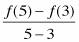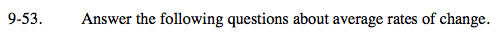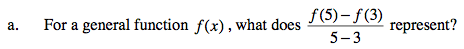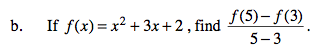### Home > AC > Chapter 16 > Lesson 16.9.1.4 > Problem9-53

9-53.
1. Answer the following questions about average rates of change. Homework Help ✎

1. For a general function f(x), what doesrepresent?

2. If f(x) = x2 + 3x + 2, find.The average rate of change of f on the interval [3,5].
It is the slope of the line between those 2 points.11